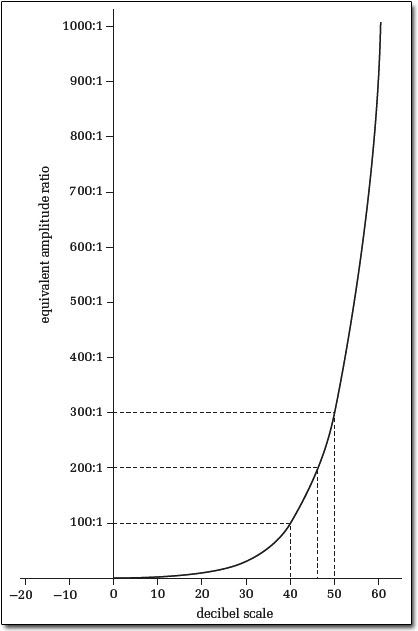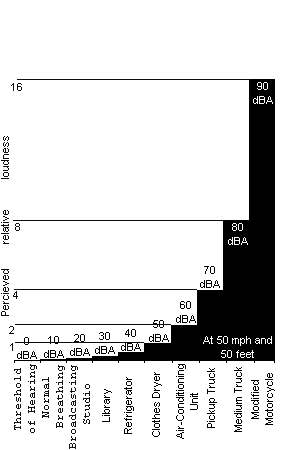1. ## Decibels

we all know our pipes are loud but how loud is LOUDok for starts db's are not linear there logarythmic and as i aint no mathemeticain heres a re few scales for you to peruseReply With Quote

2. So where's the kids with the stereo that is about to break their windows and sounds like crap on that chart?Reply With Quote

3. between pick up truck and modified motorcycle LOL 90db is a lot less than my bike mine db at 108dbReply With Quote

4.Originally Posted by Hobbitbetween pick up truck and modified motorcycle LOL 90db is a lot less than my bike mine db at 108dbYeah, don't know what mine is, probably lower than 90, but there are quite a few around here that would be way off that chart..Reply With Quote

5. space shuttle is 140+db !!!! rock concert around 110db, jumbo 135dbReply With Quote

6. Rosie O'Donnell = 180dbReply With Quote

7.Originally Posted by RumRunnerRosie O'Donnell = 180dbooooooooooooohh..';';';';'Reply With Quote

8. Need to add mother inlaw on there, she is around 90 to 110 dba on a god day..
But I do love my mother inlaw, ask my wife she will tell you...HEHEHEHEHE!Reply With Quote

9. Here is what I learned in school about sound and dba measurements where the sound volume doubles with just 3dba difference. Pulled the following example off internet.

Definition and examples
The decibel (dB) is used to measure sound level, but it is also widely used in electronics, signals and communication. The dB is a logarithmic unit used to describe a ratio. The ratio may be power, sound pressure, voltage or intensity or several other things. Later on we relate dB to the phon and the sone (units related to loudness). But first, to get a taste for logarithmic units, let's look at some numbers. (If you have forgotten, go to What is a logarithm?)

For instance, suppose we have two loudspeakers, the first playing a sound with power P1, and another playing a louder version of the same sound with power P2, but everything else (how far away, frequency) kept the same.

The difference in decibels between the two is defined to be

10 log (P2/P1) dB where the log is to base 10.
If the second produces twice as much power than the first, the difference in dB is
10 log (P2/P1) = 10 log 2 = 3 dB.

If the second had 10 times the power of the first, the difference in dB would be
10 log (P2/P1) = 10 log 10 = 10 dB.

If the second had a million times the power of the first, the difference in dB would be
10 log (P2/P1) = 10 log 1,000,000 = 60 dB.

This example shows one feature of decibel scales that is useful in discussing sound: they can describe very big ratios using numbers of modest size. But note that the decibel describes a ratio:Reply With Quote

10.Originally Posted by GlenaltHere is what I learned in school about sound and dba measurements where the sound volume doubles with just 3dba difference. Pulled the following example off internet.

Definition and examples
The decibel (dB) is used to measure sound level, but it is also widely used in electronics, signals and communication. The dB is a logarithmic unit used to describe a ratio. The ratio may be power, sound pressure, voltage or intensity or several other things. Later on we relate dB to the phon and the sone (units related to loudness). But first, to get a taste for logarithmic units, let's look at some numbers. (If you have forgotten, go to What is a logarithm?)

For instance, suppose we have two loudspeakers, the first playing a sound with power P1, and another playing a louder version of the same sound with power P2, but everything else (how far away, frequency) kept the same.

The difference in decibels between the two is defined to be

10 log (P2/P1) dB where the log is to base 10.
If the second produces twice as much power than the first, the difference in dB is
10 log (P2/P1) = 10 log 2 = 3 dB.

If the second had 10 times the power of the first, the difference in dB would be
10 log (P2/P1) = 10 log 10 = 10 dB.

If the second had a million times the power of the first, the difference in dB would be
10 log (P2/P1) = 10 log 1,000,000 = 60 dB.

This example shows one feature of decibel scales that is useful in discussing sound: they can describe very big ratios using numbers of modest size. But note that the decibel describes a ratio:
Is there going to be a test on this in the am? I think I am going to be sick tomorrow!Reply With Quote

Page 1 of 2 12 Last####Posting Permissions

• You may not post new threads
• You may not post replies
• You may not post attachments
• You may not edit your posts
•

HarleyTalking.com is a privately owned internet discussion forum and is not affiliated with, endorsed by, or sponsored by Harley Davidson, Inc in any way. The Harley Davidson names and logos are trademarks owned by Harley-Davidson, Inc.